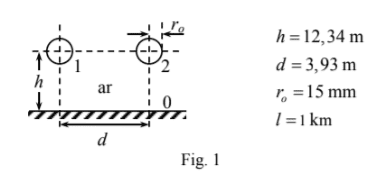# System of multiple conductors: image method

## Homework Statement

The following figure represent the traversal cut of a system with two cylindrical equal conductors of radius r0 length l at a distance d from one another and at the same distance h of a plane conductor (conductor zero). The dielectric that surrounds the conductors is the air which maximum electric field 20 kV/cm. Consider the approximation of thin conductors. By applying the image method determine the capacitance matrix of the system## Homework Equations

3. The Attempt at a Solution [/B]

So I have no idea where to start...

I now how to apply the image method to a single cylindrical conductor and a plane which would leave us to obtain:

$$\frac{2 \pi \epsilon_0 l}{\ln (\frac{h}{r_0} + \sqrt{(\frac{h}{r_0})^2 -1})} =\frac{2 \pi \epsilon_0 l}{\ln (\frac{2h}{r_0})}$$

Where I applied the fact that the conductor is thin in the last equality.
Now how do I relate this with the second conductor.
I read that this is the solution for the capacitance between each conductor and the ground plane (so the main diagonal of the matrix). But how is it valid that we "ignore" the second conductor when we calculate the capacitance between one conductor and the ground plane? Is it because the conductors are thin?

Now what about the conductance between conductor 1 and conductor 2 (C12 and C21 on the matrix)? How do we apply the image method? Do I need to consider 4 conductors? What is the form of the equipotentials? Are they still circumferences as in the case of a single conductor and a plane?

I am really confused... Can someone give hints about how to attack the problem?